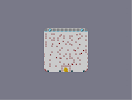### FireworksHover over the thumbnail for a full-size version.

Author Prof_Cat author:prof_cat playable survival unrated 2005-12-26 4 more votes required for a rating. \$Fireworks#Prof_Cat#none#11111111111111111111111111111111111111111111111111111111111111111111111111111111111111111111111111111111111111111111111111111111111111111111111111111111111111111111111111111111111111111111111111111111111111111111111111111111111111111111900000000061111111111110000000000011111111111100000000000111111111111000000000001111111111110000000000011111111111100000000000111111111111000000000001111111111110000000000011111111111100000000000111111111111000000000001111111111118000000000711111111111111111111111111111111111111111111111111111111111111111111111111111111111111111111111111111111111111111111111111111111111111111111111111111111111111111111111111111111111111111111111111111111111111111111111111111111111111111111|5^396,420!4^288,426,1!4^504,426,1!4^432,426,1!4^360,426,1!12^278,430!12^278,430!12^278,427!12^276,426!12^272,425!12^272,425!12^270,419!12^270,419!12^268,418!12^268,418!12^268,418!12^268,418!12^268,416!12^268,416!12^270,417!12^272,419!12^272,419!12^273,421!12^273,421!12^273,421!12^275,425!12^275,425!12^275,426!12^513,431!12^513,431!12^513,431!12^516,427!12^520,425!12^520,425!12^520,425!12^523,418!12^523,416!12^525,415!12^527,420!12^524,424!12^524,425!12^524,425!12^521,426!12^520,426!12^516,428!12^515,428!12^514,428!12^514,428!12^514,428!12^303,329!12^342,278!12^379,248!12^431,269!12^452,304!12^402,352!12^366,349!12^356,261!12^367,209!12^462,208!12^479,267!12^489,320!12^436,386!12^339,346!12^316,264!12^400,177!12^471,233!12^502,303!12^458,385!12^411,385!8^396,180,1!8^276,180,1!8^300,180,1!8^324,180,1!8^348,180,1!8^372,180,1!8^420,180,1!8^444,180,1!8^468,180,1!8^492,180,1!8^516,180,1!6^492,180,2,0,0,1!6^300,180,3,0,0,1!12^300,396!12^300,384!12^312,312!12^312,276!12^324,240!12^348,240!12^396,264!12^444,300!12^456,324!12^444,372!12^432,384!12^360,372!12^360,348!12^372,288!12^480,228!12^492,216!12^420,216!12^360,216!12^300,228!12^312,240!12^420,348!12^444,360!12^384,384!12^432,348!12^456,252!12^312,276!12^408,300!12^420,336!12^360,348!12^480,384!12^480,324!12^468,288!12^456,252!12^420,228!12^420,228!12^420,264!12^420,324!12^360,324!12^348,312!12^360,300!12^408,252!12^432,240!12^432,216!12^384,216!12^384,228!12^264,384!12^264,372!12^264,336!12^264,324!12^276,276!12^276,264!12^276,252!12^276,228!12^276,216!12^276,204!12^324,228!12^528,396!12^528,372!12^528,348!12^528,324!12^516,312!12^516,300!12^516,276!12^516,240!12^516,216!12^480,204!12^312,204!12^324,204!12^348,228!12^384,336!12^396,324!12^480,360!12^468,348!12^336,396!12^300,360!12^300,360!12^396,396!12^396,396!12^396,396!12^396,396!12^396,396!12^396,396!12^396,396!12^396,396!12^396,396!12^396,396!12^396,396!12^396,396!12^396,396!12^396,396!12^396,396!12^396,396!12^396,396!12^396,396!12^396,396!12^396,396!12^396,396!12^396,396!12^396,396!12^396,396!12^396,396!12^408,396!12^408,396!12^408,396!12^408,396!12^408,396!12^408,396!12^408,396!12^384,396!12^384,396!12^384,396!12^384,396!12^384,396!12^384,396!12^384,396!12^372,396!12^372,396!12^372,396!12^372,396!12^372,396!12^372,396!12^372,396!12^372,408!12^372,408!12^372,408!12^372,408!12^372,408!12^372,408!12^372,408!12^372,420!12^372,420!12^372,420!12^372,420!12^372,420!12^372,420!12^372,420!12^372,432!12^372,432!12^372,432!12^372,432!12^372,432!12^372,432!12^420,432!12^420,432!12^420,432!12^420,420!12^420,420!12^420,420!12^420,420!12^420,432!12^420,432!12^420,420!12^420,420!12^420,408!12^420,408!12^420,408!12^420,396!12^420,396!12^420,396!12^420,396!12^420,408!12^420,408!12^420,420!12^420,420!12^420,420!12^420,420!12^420,420!0^399,425!0^397,420!0^394,415!0^393,415!0^396,414!0^397,414!0^397,417!0^398,418!0^398,424!0^395,425!0^394,419!0^393,418!0^391,415!0^390,415!0^389,416!0^388,418!0^388,419!0^386,416!0^386,416!0^385,421!0^386,420!0^394,409!0^394,407!0^394,411!0^396,415!0^404,427!0^405,428!0^405,428!0^402,428!0^402,428!0^399,425!0^399,424!0^401,426!0^401,426!0^401,427!0^402,425!0^403,419!0^403,415!0^400,412!0^396,409!0^394,407!0^389,407!0^388,407!0^389,415!0^389,418!0^390,428!0^390,428!0^384,423!0^384,423!0^388,424# There should be a "suicide" or "explosive" category, but it is very actionpacked. Best run in debug mode for full, erm, fun.